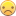## Eulidean distance in TSP

Hello,

I find here code for calculate

Traveling Salesman Problem

so I a little modified it to this:

``````proc sql;
select
a.Mesta as Mesta1, a.x as lat1, a.y as long1,
b.Mesta as Mesta2, b.x as lat2, b.y as long2,
geodist(lat1, long1, lat2, long2,'D' ) as distance
from Burma as a, Burma as b
where a.Mesta < b.Mesta;
quit;
proc optnet
loglevel   = moderate
out_nodes  = TSPTourNodes;
from    = Mesta1
to      = Mesta2
weight  = distance;
tsp
run;
%put &_OROPTNET_;
%put &_OROPTNET_TSP_;``````

Problem is, that my Dataset is in EUC_2D-norm. Could someone help me, modify the code for EUC_2D-norm?? PleaseExample of dataset:

```1 24748.3333 50840.0000
2 24758.8889 51211.9444
3 24827.2222 51394.7222
4 24904.4444 51175.0000
5 24996.1111 51548.8889
6 25010.0000 51039.4444
7 25030.8333 51275.2778
8 25067.7778 51077.5000
9 25100.0000 51516.6667
10 25103.3333 51521.6667
11 25121.9444 51218.3333
12 25150.8333 51537.7778
13 25158.3333 51163.6111
14 25162.2222 51220.8333
15 25167.7778 51606.9444```

Thank you1 ACCEPTED SOLUTION

Accepted Solutions

## Re: Eulidean distance in TSP

round(euclid(lat1-lat2, long1-long2))

or

round(sqrt((lat1-lat2)**2 + (long1-long2)**2))

9 REPLIES 9

## Re: Eulidean distance in TSP

I don't work in OR but I'm going to make some guesses.

Your starting data is in a similar form so the difference would only be in the distance calculation.

Since your using Euclidean distance replace the geodist formula with the Euclidean distance formula. There's probably even a function to do so.

## Re: Eulidean distance in TSP

Note that EUC_2D is rounded Euclidean distance.  You can replace the GEODIST call with an expression that uses the ROUND and SQRT (or ROUND and EUCLID) functions.

## Re: Eulidean distance in TSP

I used EUCLID functions, but my result is still wrong. Not even close to right result.Do not know, what I am doing wrong.

## Re: Eulidean distance in TSP

Please show the code and log.

## Re: Eulidean distance in TSP

Code:

``````proc sql;
select
a.Mesta as Mesta1, a.x as lat1, a.y as long1,
b.Mesta as Mesta2, b.x as lat2, b.y as long2,
euclid(lat1, long1, lat2, long2) as distance
from Burma as a, Burma as b
where a.Mesta < b.Mesta;
quit;
proc optnet
loglevel   = moderate
out_nodes  = TSPTourNodes;
from    = Mesta1
to      = Mesta2
weight  = distance;
tsp
run;
%put &_OROPTNET_;
%put &_OROPTNET_TSP_;``````

Log:

```1                                                          The SAS System                               21:36 Sunday, March 13, 2016

1          ;*';*";*/;quit;run;
2          OPTIONS PAGENO=MIN;
4          %LET _CLIENTPROJECTPATH='';
5          %LET _CLIENTPROJECTNAME='';
6          %LET _SASPROGRAMFILE=;
7
8          ODS _ALL_ CLOSE;
9          OPTIONS DEV=ACTIVEX;
10         GOPTIONS XPIXELS=0 YPIXELS=0;
11         FILENAME EGSR TEMP;
12         ODS tagsets.sasreport13(ID=EGSR) FILE=EGSR
13             STYLE=HtmlBlue
14             STYLESHEET=(URL="file:///C:/SAS/SASHome/SASEnterpriseGuide/6.1/Styles/HtmlBlue.css")
15             NOGTITLE
16             NOGFOOTNOTE
17             GPATH=&sasworklocation
18             ENCODING=UTF8
19             options(rolap="on")
20         ;
NOTE: Writing TAGSETS.SASREPORT13(EGSR) Body file: EGSR
21
22         GOPTIONS ACCESSIBLE;
23         proc sql;
25            select
26               a.Mesta as Mesta1, a.x as lat1, a.y as long1,
27               b.Mesta as Mesta2, b.x as lat2, b.y as long2,
28               euclid(lat1, long1, lat2, long2) as distance
29               from Burma as a, Burma as b
30               where a.Mesta < b.Mesta;
NOTE: The execution of this query involves performing one or more Cartesian product joins that can not be optimized.
NOTE: Table WORK.BURMADIST created, with 18721 rows and 7 columns.

31         quit;
NOTE: PROCEDURE SQL used (Total process time):
real time           0.03 seconds
cpu time            0.03 seconds

32         proc optnet
33            loglevel   = moderate
35            out_nodes  = TSPTourNodes;
37               from    = Mesta1
38               to      = Mesta2
39               weight  = distance;
40            tsp
42         run;

NOTE: ------------------------------------------------------------------------------------------------------------------------------
NOTE: ------------------------------------------------------------------------------------------------------------------------------
NOTE: Running OPTNET version 13.1.
NOTE: ------------------------------------------------------------------------------------------------------------------------------
NOTE: ------------------------------------------------------------------------------------------------------------------------------
2                                                          The SAS System                               21:36 Sunday, March 13, 2016

NOTE: Data input used 0.05 (cpu: 0.04) seconds.
NOTE: Building the input graph storage used 0.02 (cpu: 0.02) seconds.
NOTE: The input graph storage is using 2.3 MBs of memory.
NOTE: The number of nodes in the input graph is 194.
NOTE: The number of links in the input graph is 18721.
NOTE: ------------------------------------------------------------------------------------------------------------------------------
NOTE: ------------------------------------------------------------------------------------------------------------------------------
NOTE: Processing the traveling salesman problem.
NOTE: The initial TSP heuristics found a tour with cost 15722636.846 using 4.23 (cpu: 4.12) seconds.
NOTE: The MILP presolver value NONE is applied.
NOTE: The MILP solver is called.
Node  Active    Sols    BestInteger      BestBound      Gap    Time
0       1       1       15722637       15722636    0.00%       0
0       1       1       15722637       15722636    0.00%       0
NOTE: Optimal within relative gap.
NOTE: Objective = 15722636.846.
NOTE: Processing the traveling salesman problem used 5.23 (cpu: 4.99) seconds.
NOTE: ------------------------------------------------------------------------------------------------------------------------------
NOTE: ------------------------------------------------------------------------------------------------------------------------------
NOTE: Creating nodes data set output.
NOTE: Creating traveling salesman data set output.
NOTE: Data output used 0.00 (cpu: 0.01) seconds.
NOTE: ------------------------------------------------------------------------------------------------------------------------------
NOTE: ------------------------------------------------------------------------------------------------------------------------------
NOTE: The data set WORK.TSPTOURNODES has 194 observations and 2 variables.
NOTE: The data set WORK.TSPTOURLINKS has 194 observations and 3 variables.
NOTE: PROCEDURE OPTNET used (Total process time):
real time           5.33 seconds
cpu time            5.35 seconds

43         %put &_OROPTNET_;
STATUS=OK  TSP=OPTIMAL_RGAP
44         %put &_OROPTNET_TSP_;
STATUS=OPTIMAL_RGAP  OBJECTIVE=15722636.846  RELATIVE_GAP=4.703E-8  ABSOLUTE_GAP=0.7394683491  PRIMAL_INFEASIBILITY=0
BOUND_INFEASIBILITY=0  INTEGER_INFEASIBILITY=0  BEST_BOUND=15722636.106  NODES=1  ITERATIONS=544  CPU_TIME=4.99  REAL_TIME=5.23
45
46         GOPTIONS NOACCESSIBLE;
48         %LET _CLIENTPROJECTPATH=;
49         %LET _CLIENTPROJECTNAME=;
50         %LET _SASPROGRAMFILE=;
51
52         ;*';*";*/;quit;run;
53         ODS _ALL_ CLOSE;
54
55
56         QUIT; RUN;
57         ```

Optimal Value should be:  9352

## Re: Eulidean distance in TSP

Hint 1:

The arguments for the EUCLID function are the numbers to be squared:

http://support.sas.com/documentation/cdl/en/syntaxidx/68719/HTML/default/index.htm#/documentation/cd...

Hint 2:

You also need to use the ROUND function.

## Re: Eulidean distance in TSP

I added ROUND function and SQRT, but probably in wrong place, because I still have incorrect result.

``````proc sql;
select
a.Mesta as Mesta1, a.x as lat1, a.y as long1,
b.Mesta as Mesta2, b.x as lat2, b.y as long2,
sqrt(round(euclid(round(lat1,1), round(long1,1),round(lat2,1), round(long2,1)),1)) as distance
from Burma as a, Burma as b
where a.Mesta < b.Mesta;
quit;
proc optnet
loglevel   = moderate
out_nodes  = TSPTourNodes;
from    = Mesta1
to      = Mesta2
weight  = distance;
tsp
run;
%put &_OROPTNET_;
%put &_OROPTNET_TSP_;``````

## Re: Eulidean distance in TSP

round(euclid(lat1-lat2, long1-long2))

or

round(sqrt((lat1-lat2)**2 + (long1-long2)**2))

## Re: Eulidean distance in TSP

Thank you very much
Discussion stats
• 9 replies
• 1565 views
• 1 like
• 3 in conversation